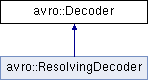Avro C++
avro::Decoder Class Reference

Decoder is an interface implemented by every decoder capable of decoding Avro data. More...

`#include <Decoder.hh>`

Inheritance diagram for avro::Decoder:List of all members.

## Public Member Functions

virtual void init (InputStream &is)=0
All future decoding will come from is, which should be valid until replaced by another call to init() or this Decoder is destructed.
virtual void decodeNull ()=0
Decodes a null from the current stream.
virtual bool decodeBool ()=0
Decodes a bool from the current stream.
virtual int32_t decodeInt ()=0
Decodes a 32-bit int from the current stream.
virtual int64_t decodeLong ()=0
Decodes a 64-bit signed int from the current stream.
virtual float decodeFloat ()=0
Decodes a single-precision floating point number from current stream.
virtual double decodeDouble ()=0
Decodes a double-precision floating point number from current stream.
std::string decodeString ()
Decodes a UTF-8 string from the current stream.
virtual void decodeString (std::string &value)=0
Decodes a UTF-8 string from the stream and assigns it to value.
virtual void skipString ()=0
Skips a string on the current stream.
std::vector< uint8_t > decodeBytes ()
Decodes arbitray binary data from the current stream.
virtual void decodeBytes (std::vector< uint8_t > &value)=0
Decodes arbitray binary data from the current stream and puts it in value.
virtual void skipBytes ()=0
Skips bytes on the current stream.
std::vector< uint8_t > decodeFixed (size_t n)
Decodes fixed length binary from the current stream.
virtual void decodeFixed (size_t n, std::vector< uint8_t > &value)=0
Decodes a fixed from the current stream.
virtual void skipFixed (size_t n)=0
Skips fixed length binary on the current stream.
virtual size_t decodeEnum ()=0
Decodes enum from the current stream.
virtual size_t arrayStart ()=0
Start decoding an array. Returns the number of entries in first chunk.
virtual size_t arrayNext ()=0
Returns the number of entries in next chunk. 0 if last.
virtual size_t skipArray ()=0
Tries to skip an array.
virtual size_t mapStart ()=0
Start decoding a map. Returns the number of entries in first chunk.
virtual size_t mapNext ()=0
Returns the number of entries in next chunk. 0 if last.
virtual size_t skipMap ()=0
Tries to skip a map.
virtual size_t decodeUnionIndex ()=0
Decodes a branch of a union. The actual value is to follow.

## Detailed Description

Decoder is an interface implemented by every decoder capable of decoding Avro data.

## Member Function Documentation

 virtual void avro::Decoder::decodeBytes ( std::vector< uint8_t > & value ) ` [pure virtual]`

Decodes arbitray binary data from the current stream and puts it in value.

 std::vector avro::Decoder::decodeFixed ( size_t n ) ` [inline]`

Decodes fixed length binary from the current stream.

Parameters:
 [in] n The size (byte count) of the fixed being read.
Returns:
The fixed data that has been read. The size of the returned vector is guaranteed to be equal to `n`.
 virtual void avro::Decoder::decodeFixed ( size_t n, std::vector< uint8_t > & value ) ` [pure virtual]`

Decodes a fixed from the current stream.

Parameters:
 [in] n The size (byte count) of the fixed being read. [out] value The value that receives the fixed. The vector will be size-adjusted based on the fixed's size.
 virtual void avro::Decoder::init ( InputStream & is ) ` [pure virtual]`

All future decoding will come from is, which should be valid until replaced by another call to init() or this Decoder is destructed.

 virtual size_t avro::Decoder::skipArray ( ) ` [pure virtual]`

Tries to skip an array.

If it can, it returns 0. Otherwise it returns the number of elements to be skipped. The client should skip the individual items. In such cases, skipArray is identical to arrayStart.

 virtual size_t avro::Decoder::skipMap ( ) ` [pure virtual]`

Tries to skip a map.

If it can, it returns 0. Otherwise it returns the number of elements to be skipped. The client should skip the individual items. In such cases, skipMap is identical to mapStart.

The documentation for this class was generated from the following file: